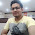### C Language program to calculate factorial of a number using for loop

Introduction: In this article i am going to write/create a program to find/get/calculate factorial of entered number using For loop in C language. In previous articles i explained the programs to Print table of entered number using For loop and Find greatest of three numbers using nested if else and Get number of days in month and year using else if ladder and Calculate sum,average and division of marks using else-if ladderFind month name corresponding to number using switch case .

Description:  To calculate factorial of number, simply multiply  from 1 to that number or that number to 1 i.e. the factorial of a number 'n' is the product of all number from 1 up to the number 'n' or from 'n' up to 1 and it is denoted by n!.

Rule: n! = n*(n-1)!

So 1! = 1
2! = 2×1 = 2
3! = 3×2×1 = 6
4! = 4×3×2×1 = 24
5! = 5×4×3×2×1 = 120
6!=  6×5×4×3×2×1 = 720

or we can say if n=6 then factorial of 6 will be calculated as 1*2*3*4*5*6= 720 or 6*5*4*3*2*1=720.  So 6 != 720.

Implementation: Let's create a program to calculate Factorial of a number.

#include<conio.h>
#include<stdio.h>
int main()
{
unsigned long long int fact=1;
int i,n;
clrscr();
printf("Enter any positive number to calculate its factorial: ");
scanf("%d",&n);
for(i=1;i<=n;i++)
{
fact=fact*i;
}
printf("\nFactorial of %d = %llu",n,fact);
getch();
return 0;
}

Note:  We can also use
for(i=n;i>=1;i--)
{
fact=fact*i;
}
for(i=1;i<=n;i++)
{
fact=fact*i;
}
in the above program to calculate the factorial.
• Now Run the program using Ctrl+F9
Output:

Enter any positive number to calculate its factorial: 6

Factorial of 6 = 720

Now over to you:
"If you like my work; you can appreciate by leaving your comments, hitting Facebook like button, following on Google+, Twitter, Linked in and Pinterest, stumbling my posts on stumble upon and subscribing for receiving free updates directly to your inbox . Stay tuned and stay connected for more technical updates."
Previous
Next Post »

Unknown